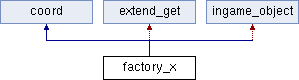Simutrans-Squirrel-API
factory_x Class Reference
Inheritance diagram for factory_x:## Public Member Functions

factory_x (integer x, integer y)

bool is_valid ()

array< coordget_consumers ()

array< coordget_suppliers ()

string get_name ()

string set_name (string)

array< integer > get_production ()

array< integer > get_power ()

array< integer > get_boost_electric ()

array< integer > get_boost_pax ()

array< integer > get_boost_mail ()

array< integer > get_pax_generated ()

array< integer > get_pax_departed ()

array< integer > get_pax_arrived ()

array< integer > get_mail_generated ()

array< integer > get_mail_departed ()

array< integer > get_mail_arrived ()

array< coordget_tile_list ()

array< halt_xget_halt_list ()

## Public Attributes

table< factory_production_xinput

table< factory_production_xoutputPrivate Member Functions inherited from coord
coord (int x, int y)

coord operator+ (coord other)

coord operator- (coord other)

coord operator- ()

coord operator* (integer fac)

coord operator/ (integer fac)

string _tostring ()

string href (string text)

dir to_dir ()Private Attributes inherited from coord
integer x
x-coordinate

integer y
y-coordinate

## Detailed Description

Class to access information about factories. Identified by coordinate.

Definition at line 36 of file api_factory.cc.

## Constructor & Destructor Documentation

 factory_x::factory_x ( integer x, integer y )

Constructor.

Parameters
 x x-coordinate y y-coordinate

## Member Function Documentation

 array factory_x::get_boost_electric ( )

Get monthly statistics of production boost due to electric power supply.

Returns
array, index  corresponds to current month
 array factory_x::get_boost_mail ( )

Get monthly statistics of production boost due to arrived mail.

Returns
array, index  corresponds to current month
 array factory_x::get_boost_pax ( )

Get monthly statistics of production boost due to arrived passengers.

Returns
array, index  corresponds to current month
 array factory_x::get_consumers ( )

Get list of consumers of this factory.

Returns
array of coordinates of consumers
 array factory_x::get_halt_list ( )

Get list of all halts that serve this this factory.

Returns
array of tile_x objects
 array factory_x::get_mail_arrived ( )

Get monthly statistics of arrived mail.

Returns
array, index  corresponds to current month
 array factory_x::get_mail_departed ( )

Get monthly statistics of departed mail.

Returns
array, index  corresponds to current month
 array factory_x::get_mail_generated ( )

Get monthly statistics of generated mail.

Returns
array, index  corresponds to current month
 string factory_x::get_name ( )

Get (translated or custom) name of factory.

Returns
name
 array factory_x::get_pax_arrived ( )

Get monthly statistics of arrived passengers.

Returns
array, index  corresponds to current month
 array factory_x::get_pax_departed ( )

Get monthly statistics of departed passengers.

Returns
array, index  corresponds to current month
 array factory_x::get_pax_generated ( )

Get monthly statistics of generated passengers.

Returns
array, index  corresponds to current month
 array factory_x::get_power ( )

Get monthly statistics of power consumption/production.

Returns
array, index  corresponds to current month
 array factory_x::get_production ( )

Get monthly statistics of production.

Returns
array, index  corresponds to current month
 array factory_x::get_suppliers ( )

Get list of consumers of this factory.

Returns
array of coordinates of suppliers
 array factory_x::get_tile_list ( )

Get list of all tiles occupied by buildings belonging to this factory.

Returns
array of tile_x objects
 bool factory_x::is_valid ( )
Returns
if object is still valid.

## Member Data Documentation

 table factory_x::input

Table containing input/consumption slots. Entries can be retrieved by raw name of the good (as defined in the associated pak-file).

Entries are of type factory_production_x.

To print a list of all available goods names use this example:

foreach(key,value in input) {
// print raw name of the good
print("Input slot key: " + key)
// print current storage
print("Input slot storage: " + value.get_storage())
}

To catch the output of this example see Logging and error handling.

Definition at line 61 of file api_factory.cc.

 table factory_x::output

Table containing output/production slots. Entries can be retrieved by raw name of the good (as defined in the associated pak-file).

Entries are of type factory_production_x.

For an example to retrieve the list of goods, see factory_x::input.

Definition at line 70 of file api_factory.cc.

The documentation for this class was generated from the following file: